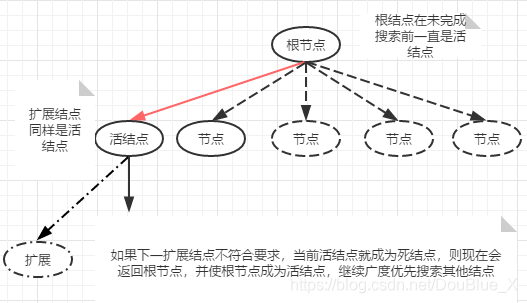# 算法第五章作业

### 一.问题描述

7-2 最小重量机器设计问题 (25 分)

### 二.算法描述

void BackTrack(int t)
{
if(t==n)//到达最后一层
{
for(int i=1; i<=m; i++)//遍历所有叶子节点
{
cw+=w[t][i];
cc+=c[t][i];
x[t]=i;
if(cc<=max_c&&cw<bestw)//若费用不超过最大费用并且重量小于之前的最优解
{
//更新最优解
bestw=cw;
for(int j=1; j<=n; j++)
bestx[j]=x[j];
}
cc-=c[t][i];//返回上一层
cw-=w[t][i];
x[t]=0;
}
}
else//未到达最后一层
{
for(int i=1; i<=m; i++)//遍历该层节点
{
x[t]=i;
cw+=w[t][i];
cc+=c[t][i];
if(cc<max_c&&cw<bestw)//费用小于最小费用重量小于最优解时进入下一层
{
BackTrack(t+1);
}
cc-=c[t][i];//返回上一层
cw-=w[t][i];
x[t]=0;

}
}
}

1.回溯法

2.算法解释

### 四.回溯法心得体会、

void Backtrack(int t)
{//以深度优先的方式遍历第t层中的某棵子树
if(t>n) { Output(x); return; }
if (……)
{  x[t]=1; Backtrack(t+1); }
if (……)
{  x[t]=0; Backtrack(t+1); }
}posted @ 2021-12-14 21:41  ammmm  阅读(38)  评论(0编辑  收藏  举报